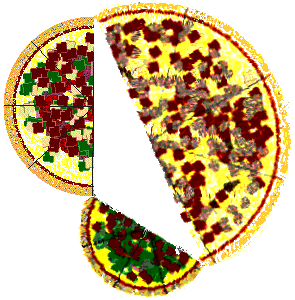Date: Wed, 19 Feb 1997 19:21:05 -0600 (CST)
Sender: Sherryle
Subject: Right Triangles

Name: Sherryle

Level: Secondary

Question:
I am a graduating senior presently teaching geometry as part of my student teaching. I will do my CUP on Right Triangles and Pythagorean theorem. I am looking for a fun activity as part of my unit plan. Can you help? I have so little time. Please help.
S Mathis.

Hi Sherryle

An interesting activity is to play the Pythagorean Theorem backwards (from the way we usually do).

Take three circles (fictional pizzas) two medium one large. To determine whether the large pizza is bigger (has more area) Cut the circles in half, put the two medium pizzas down as two edges of a triangle and the large as the third:Measure the angle between the two medium pizzas: (a) if this angle is > 90 degree, the large has more area; (b) if this angle is = 90 degrees, the areas are equal; (c) if this angle is < 90 degrees, the two mediums have more area. You can also reverse this: put down the two mediums with a right angle, then measure the hypotenuse to determine the size of an equivalent large pizza. This particular process, for 'adding areas' was precisely what the Greeks used the theorem for: Given two squares, use ruler and compass to draw a square whose area is the sum of the areas of these squares! After all, they trusted ruler and compass constructions more that they trusted 'numbers'!

Walter Whiteley

Pizzas supplied by The Internet Pizza Server

Go to Math Central